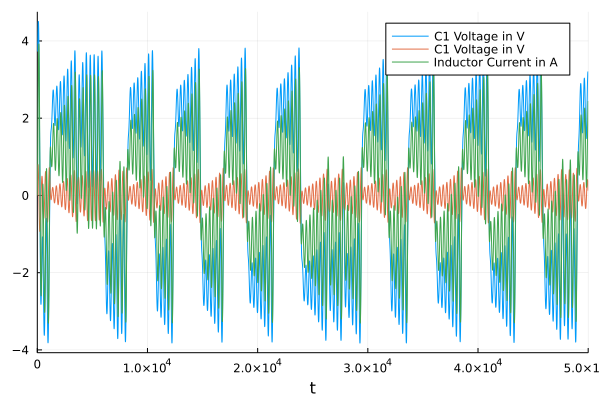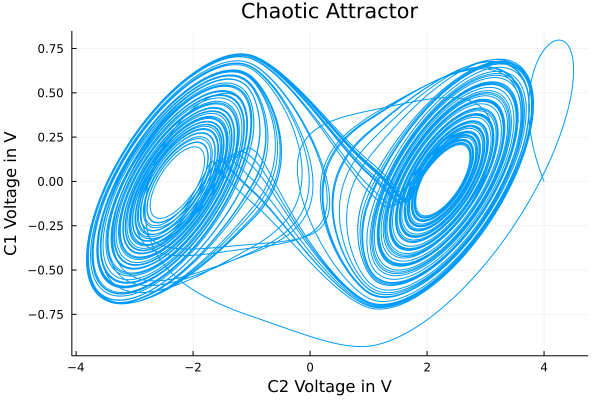# Custom Component

In this tutorial the creation of a custom component is demonstrated via the Chua's circuit. The circuit is a simple circuit that shows chaotic behaviour. Except for a non-linear resistor every other component already is part of ModelingToolkitStandardLibrary.Electrical.

First we need to make some imports.

using ModelingToolkit
using ModelingToolkitStandardLibrary.Electrical
using ModelingToolkitStandardLibrary.Electrical: OnePort
using OrdinaryDiffEq
using IfElse: ifelse
using Plots

## Custom Component

Now the custom component can be defined. The Modelica implementation of the NonlinearResistor looks as follows:

model NonlinearResistor "Chua's resistor"
extends Interfaces.OnePort;

parameter SI.Conductance Ga "conductance in inner voltage range";
parameter SI.Conductance Gb "conductance in outer voltage range";
parameter SI.Voltage Ve "inner voltage range limit";
equation
i = if (v < -Ve) then Gb*(v + Ve) - Ga*Ve else if (v > Ve) then Gb*(v - Ve) + Ga*Ve else Ga*v;
end NonlinearResistor;

this can almost be directly translate it to the syntax of ModelingToolkit.

@parameters t

function NonlinearResistor(;name, Ga, Gb, Ve)
@named oneport = OnePort()
@unpack v, i = oneport
pars = @parameters Ga=Ga Gb=Gb Ve=Ve
eqs = [
i ~ ifelse(v < -Ve,
Gb*(v + Ve) - Ga*Ve,
ifelse(v > Ve,
Gb*(v - Ve) + Ga*Ve,
Ga*v,
),
)
]
extend(ODESystem(eqs, t, [], pars; name=name), oneport)
end

### Explanation

All components in ModelingToolkit are created via a function that serves as the constructor and returns some form of system, in this case a ODESystem. Since the non-linear resistor is essentially a standard electrical component with two ports, we can extend from the OnePort component of the library.

@named oneport = OnePort()

This creates a OnePort with the name = :oneport. For easier notation we can unpack the states of the component

@unpack v, i = oneport

It might be a good idea to create parameters for the constants of the NonlinearResistor.

pars = @parameters Ga=Ga Gb=Gb Ve=Ve

The syntax looks funny but it simply creates symbolic parameters with the name Ga where it's default value is set from the function's argument Ga. While this is not strictly necessary it allows the user to remake the problem easily with different parameters or allow for auto-tuning or parameter optimization without having to do all costly steps that may be involved with building and simplifying a model. The non-linear (in this case piece-wise constant) equation for the current can be implemented using IfElse.ifelse. Finally, the created oneport component is extended with the created equations and parameters. In this case no extra state variables are added, hence an empty vector is supplied. The independent variable t needs to be supplied as second argument.

extend(ODESystem(eqs, t, [], pars; name=name), oneport)

## Building the Model

The final model can now be created with the components from the library and the new custom component.

@named L = Inductor(L=18)
@named Ro = Resistor(R=12.5e-3)
@named G = Conductor(G=0.565)
@named C1 = Capacitor(C=10, v_start=4)
@named C2 = Capacitor(C=100)
@named Nr = NonlinearResistor(
Ga = -0.757576,
Gb = -0.409091,
Ve=1)
@named Gnd = Ground()

connections = [
connect(L.p, G.p)
connect(G.n, Nr.p)
connect(Nr.n, Gnd.g)
connect(C1.p, G.n)
connect(L.n, Ro.p)
connect(G.p, C2.p)
connect(C1.n, Gnd.g)
connect(C2.n, Gnd.g)
connect(Ro.n, Gnd.g)
]

@named model = ODESystem(connections, t, systems=[L, Ro, G, C1, C2, Nr])

## Simulating the Model

Now the model can be simulated. First structural_simplify is called on the model and a ODEProblem is build from the result. Since the initial voltage of the first capacitor was already specified via v_start, no initial condition is given and an empty pair is supplied.

sys = structural_simplify(model)
prob = ODEProblem(sys, Pair[], (0, 5e4), saveat=0.01)
sol = solve(prob, Rodas4())

Plots.plot(sol[C1.v], sol[C2.v], title="Chaotic Attractor", label="", ylabel="C1 Voltage in V", xlabel="C2 Voltage in V")
Plots.savefig("chua_phase_plane.png")

Plots.plot(sol; vars=[C1.v, C2.v, L.i], labels=["C1 Voltage in V" "C1 Voltage in V" "Inductor Current in A"])
Plots.savefig("chua.png")